# Conversion miles per hour to meters per minute, mph to m/min.

The conversion factor is 26.822400010245; so 1 mile per hour = 26.822400010245 meters per minute. In other words, the value in mph multiply by 26.822400010245 to get a value in m/min. The calculator gives the answer to the questions: 120 mph is how many m/min? or change mph to m/min. Convert mph to m/min.

## Conversion result:

### 1 mph = 26.8224 m/min

1 mile per hour is 26.8224 meters per minute.

mph

### Conversion of a velocity unit in word math problems and questions

• Avg speed of flight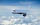The student's vice adventure had a 2,367 km flight. If their travel time was 2 hours and 56 minutes, what was their average speed in kilometers per hour?
• TouristTourist walk at 4.1 km/h. Every 5 km have 20-minute break. How many kilometers passed, when started at 4:40 and finished at 10:30?
• Speed of sound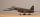The average speed of sound is 330 meters per second. Estimate how long it will take to hear the church bell 1 km away. Calculate the distance from what would hear sound after 10 seconds.
• Last wagon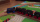A 200 m long train passes through the 700 m long tunnel so that a time of 1 minute elapses from the entry of the locomotive into the tunnel to the exit of the last wagon from the tunnel. Find the speed of the train.
• A filterIt is a pool with a volume of 3500 liters. The filter filters at 4m cubic per hour. How many minutes would it filter the entire pool?
• Cyclists and walkersA group of tourists started at 8:00 at a speed 4 km/h walk. At half-past ten, another group started on a bike and caught up with a group of tourists at 10:50. What was the average speed of cyclists?
• DecellerationIn a braking test, a vehicle that travels at 51 km/h stops in 5 s. What is the acceleration?
• Average speedWhen the bus stops at a bus stop driving average speed is 45 km/h. If it did not stop, it would drive at a speed of 54 km/h. How many minutes of every hour does it spend at stops?
• Friction coefficientWhat is the weight of a car when it moves on a horizontal road at a speed of v = 50 km/h at engine power P = 7 kW? The friction coefficient is 0.07From the junction of two streets that are perpendicular to each other, two cyclists (each on another street) walked out. One ran 18 km/h and the second 24 km/h. How are they away from a) 6 minutes, b) 15 minutes?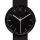What angle is betwenn hands on Clock Tower when show 17 hours and 35 minutes?The speed of the mail train is 1370 meters per minute. Express it in miles per hour, correct to three significant figures. Use the following facts to convert this units: 1 meter =39.37 inches, 1 mile = 1609 m, 1 hour = 60 minutes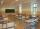George pass on the way to school distance 200 meters in 165 seconds. What is the average walking speed in m/s and km/h?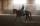Knight passed 13 km long track in 26 minutes, what was his average speed?What is the average speed of a cycle traveling at 20 km in 60 minutes in km/h?